Electric Forces and Fields Chapter 17 Section 17

• Slides: 20Electric Forces and Fields Chapter 17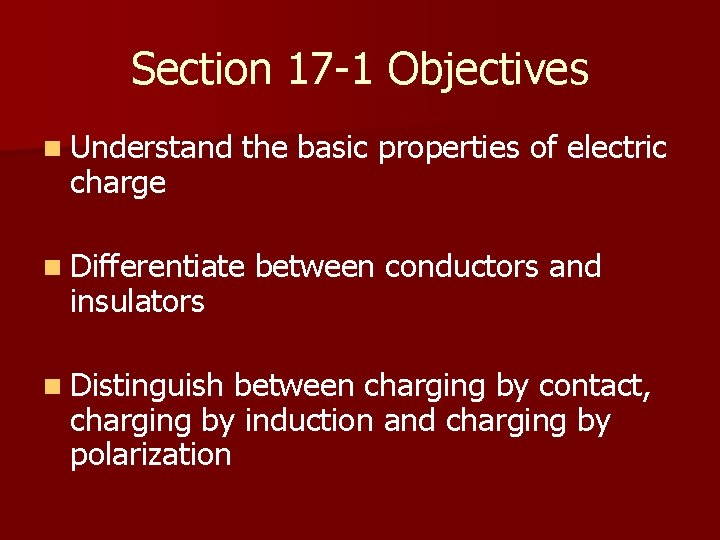Section 17 -1 Objectives n Understand charge the basic properties of electric n Differentiate insulators n Distinguish between conductors and between charging by contact, charging by induction and charging by polarizationElectric Charge What’s happening to this little girl?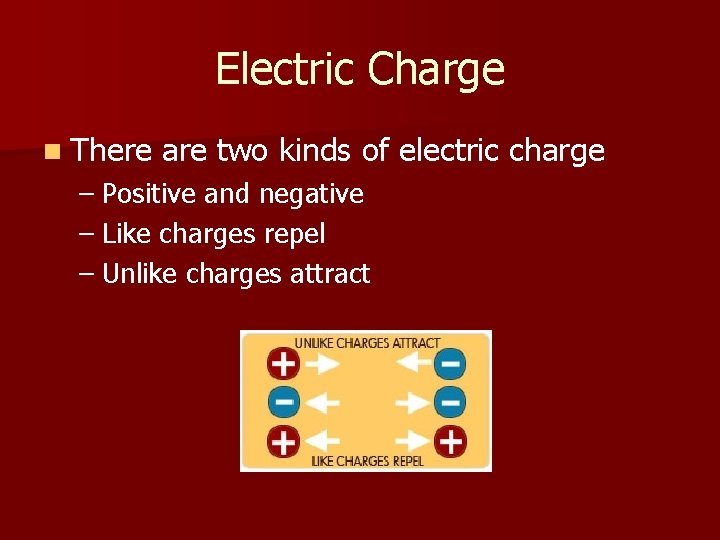Electric Charge n There are two kinds of electric charge – Positive and negative – Like charges repel – Unlike charges attractElectric Charge n Electric charge is conserved!! – What else is conserved? ? § Mass, Energy, Momentum – Electric charge cannot be created or destroyed but it can be transferred – Example: Transferring electrons from someone’s hair to a balloon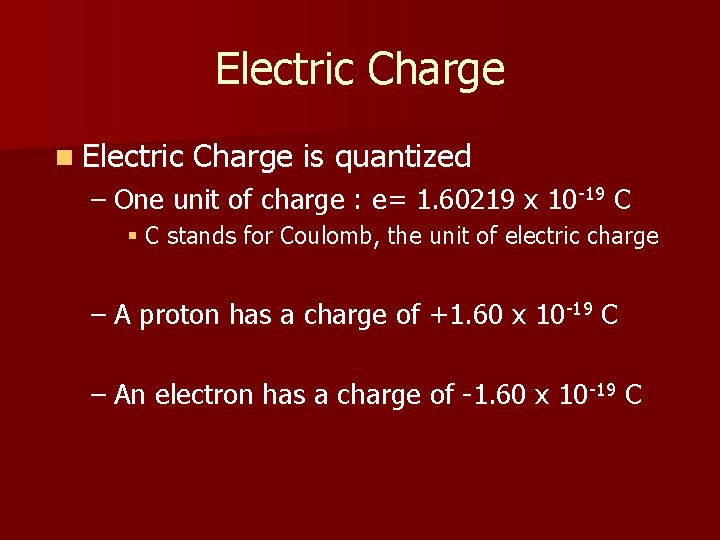Electric Charge n Electric Charge is quantized – One unit of charge : e= 1. 60219 x 10 -19 C § C stands for Coulomb, the unit of electric charge – A proton has a charge of +1. 60 x 10 -19 C – An electron has a charge of -1. 60 x 10 -19 C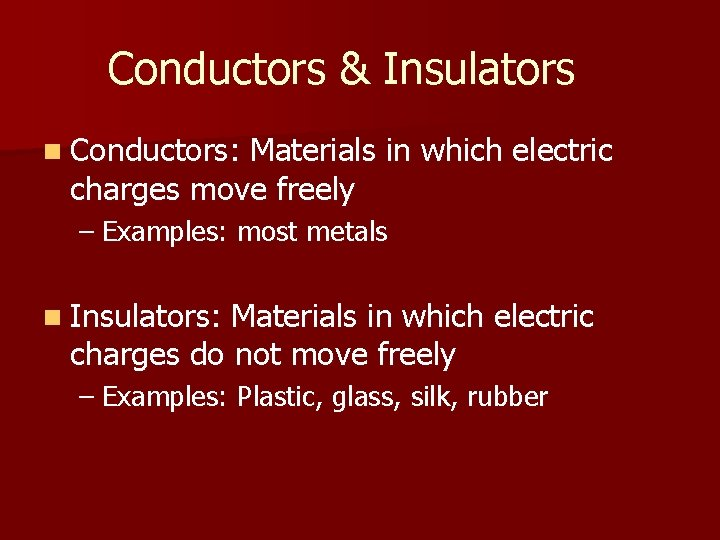Conductors & Insulators n Conductors: Materials in which electric charges move freely – Examples: most metals n Insulators: Materials in which electric charges do not move freely – Examples: Plastic, glass, silk, rubberCharging by contact n The two objects are rubbed together and electrons are transferred from one to the other – electrons from the fur are transferred to the rod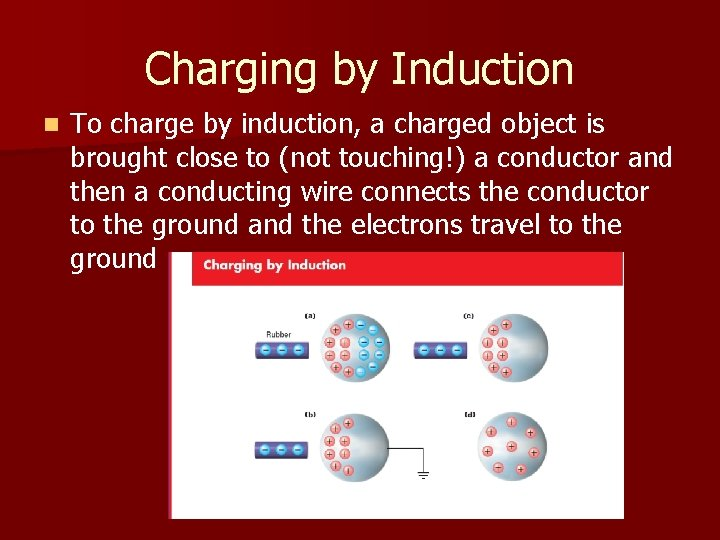Charging by Induction n To charge by induction, a charged object is brought close to (not touching!) a conductor and then a conducting wire connects the conductor to the ground and the electrons travel to the ground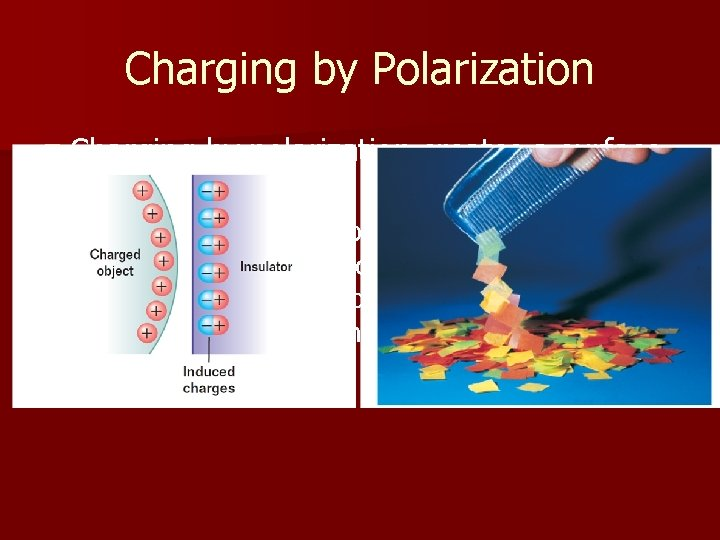Charging by Polarization n Charging charge by polarization creates a surface – A charged object is brought close to an insulator and the electrons and protons realign themselves to create one side that is more positive and one that is more negativeSection 17. 2 Objectives n Calculate electric force using coulomb’s law n Compare electric force with gravitational force n Apply the superposition principle to find the resultant force on a charge and to find the position at which the net force on a charge is zeroCoulomb’s Law n Coulomb’s Law describes the mathematical relationship between electric force, distance and electric charge for two objects k. C= 8. 99 x 109 Nm 2 C 2 Electric force= Coulomb’s Constant x (charge 1)(charge 2) distance 2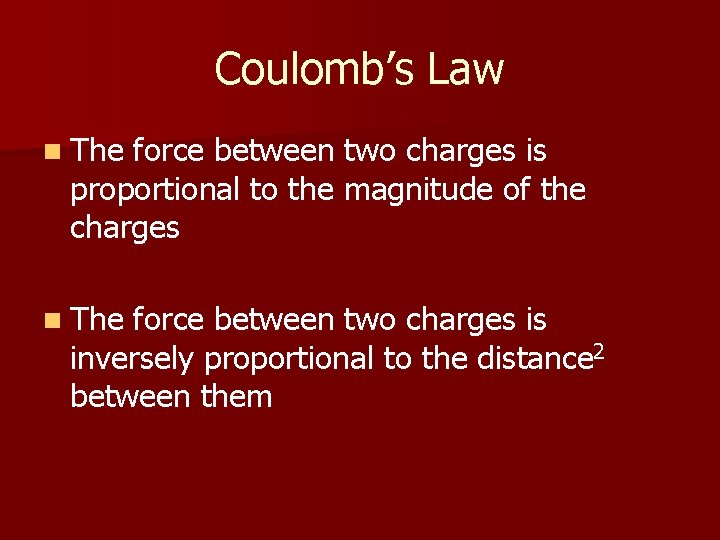Coulomb’s Law n The force between two charges is proportional to the magnitude of the charges n The force between two charges is inversely proportional to the distance 2 between themCoulomb’s Law n Remember that force is a vector! – For problems involving two charges, the direction is either “attractive” or “repulsive” – i. e. the direction of the force between a positive charge and negative charge is attractive and the direction of the force between two negative charges is repulsive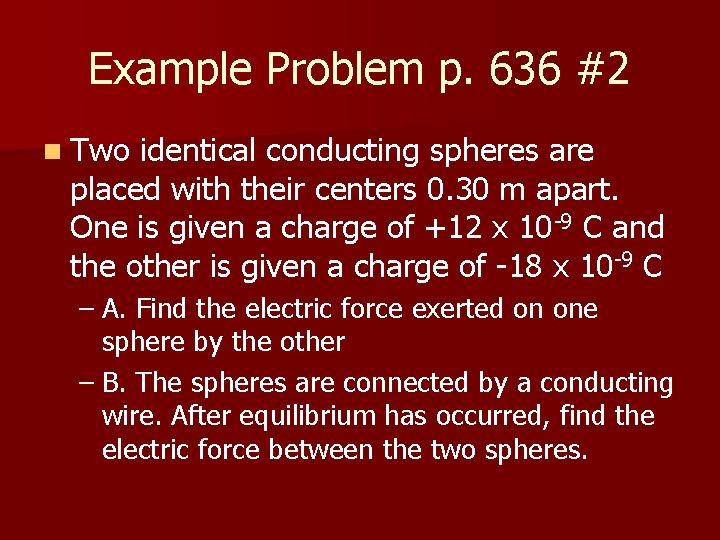Example Problem p. 636 #2 n Two identical conducting spheres are placed with their centers 0. 30 m apart. One is given a charge of +12 x 10 -9 C and the other is given a charge of -18 x 10 -9 C – A. Find the electric force exerted on one sphere by the other – B. The spheres are connected by a conducting wire. After equilibrium has occurred, find the electric force between the two spheres.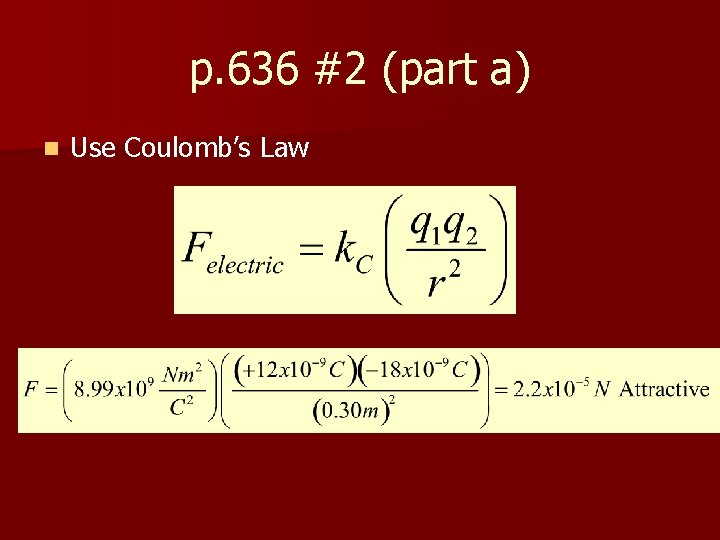p. 636 #2 (part a) n Use Coulomb’s Law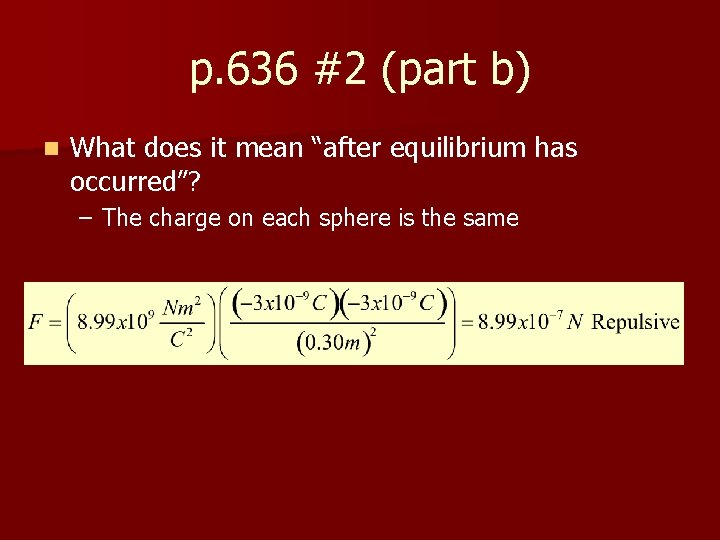p. 636 #2 (part b) n What does it mean “after equilibrium has occurred”? – The charge on each sphere is the same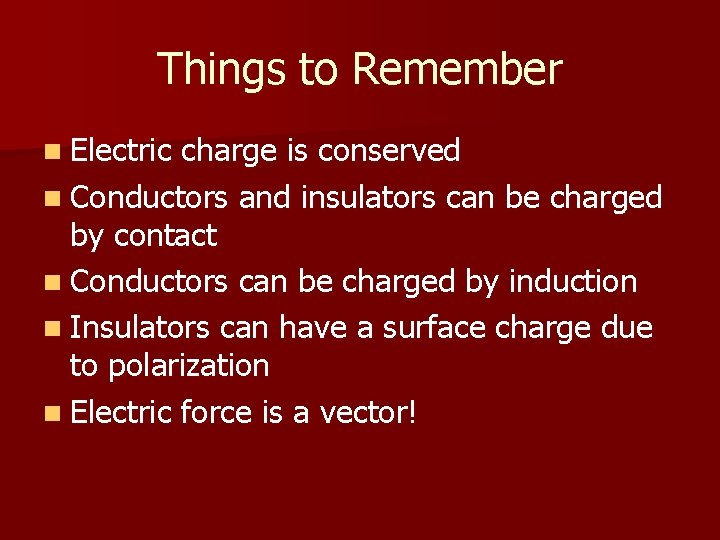Things to Remember n Electric charge is conserved n Conductors and insulators can be charged by contact n Conductors can be charged by induction n Insulators can have a surface charge due to polarization n Electric force is a vector!Sources n Balloon Hair Pic: http: //heyheyheyparty 365. blogspot. com/2 011/01/static-electricity. html n http: //startswithabang. com/? p=1643 n http: //blogs. nature. com/news/2011/06/res earchers_studying_static_ge. html